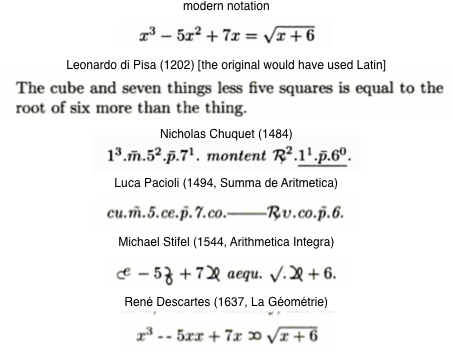# History of Mathematical Notation

I would like to see a simple example which shows how mathematical notation were evolve in time and space.

Say, consider the formula $$(x+2)^2=x^2+4{\cdot}x+4.$$ If I understand correctly, Franciscus Vieta would write something like this. (Feel free to correct me.)

## $\overline{N+2}$ quadr. æqualia $Q+N\,4+4$.

($N$ stays for unknown and $Q$ for its square.)

Can you give me the other examples?

• perhaps you might want to focus the question, in particular, to what extent is it not answered here: en.wikipedia.org/wiki/History_of_mathematical_notation Nov 28, 2015 at 12:24
• @CarloBeenakker No, Wikipedia article does not contain the answer. And example like that would be helpful for me and others. I hope that there are people here on MO who could answer. Nov 28, 2015 at 12:37
• Too broad as MO question. I think there is a consensus about the history of the use of letters in mathematics. Apart from that, each single notation has its own history. Which of these have you in mind? Nov 28, 2015 at 13:05
• Maybe Joseph Mazur's book Enlightening Symbols has an example of what you want? press.princeton.edu/titles/10204.html Nov 28, 2015 at 15:19
• This question may also be a good fit for History of Science and Mathematics.
– Danu
Nov 29, 2015 at 16:22

For a quite extensive overview with many examples, you might want to check out The origins and development of mathematical notation. I also enjoyed reading Stephen Wolfram's take on Mathematical Notation – past and future, with a great variety of illustrations.

The OP asks specifically for the evolution of one formula, "to see the big picture". Here is one example, taken from Math through the ages:I think that the most complete source is still :

We can easily find there examples regarding the evolution of "algebraic" symbolism through the modern era :

"The big picture" that can be seen in Carlo Beenakker's example is

Rhetorical (verbal); Syncopated (abbreviated words); Symbolic.

However, this well-known picture is very algebra-oriented and does not say anything about the use of notations in say geometry as discussed here " The Shaping of Deduction in Greek Mathematics: A Study in Cognitive History " or as asked here Uppercase Point Labels in High-School Diagrams. Thus, at least for me, the interesting answers to OP questions would be those that are not related to algebra.

• As an instance of the picture, the letter "e" in "et" for addition was wasted while "t" evolved to the symbol "+". Nov 29, 2015 at 8:21
• @PietroMajer what a beautiful bit of history :) Nov 29, 2015 at 9:10
• @PietroMajer, do you have evidence for this? Algebra was developed in Italian, where the word is just "e" and the "t" from Latin had been wasted. Dec 5, 2015 at 8:39
• According to Florian Cajori, the mathematical symbol + came into use in Germany, between 1450-1480. He mentions a number of manuscripts in Latin from the Dresden Library were both "+" and "et" are used somehow interchangeably both in arithmetic and grammatical use. On the grammatical side, short forms are well-known in paleography. J.L.Walthers listes 102 different forms for the conjunction "et", including "+". Dec 5, 2015 at 9:51

You might also enjoy Earliest Uses of Symbols of Operation!!

Find a quantity such that $\varsigma\;\overline{\beta}$ squared equals $$\Delta^{\Upsilon}\;\varsigma\overline{\delta}\;{\stackrel{o}{M}}\overline{\delta}$$

(My translation into English from Diophantes $\alpha\rho\iota\theta\mu\eta\tau\iota\kappa\eta$.)